## //----------------------------// ArtMatic 1 in 2 out components //----------------------------//

Introduction
Set Constant
Set Vector (Ax,Bx)
2D derivative dx,dy
Modulo & int
Integer Modulo
Cubic Clip
Math tools #
Linear motion
Circular motion
Random motion
Saw motion
Perlin noise + derivative
12 Compiled tree

## Introduction

12 components act as either "dual filters" or "motion functions". Dual filters (such as Modulo & Int) implement two related functions (such as floating point modulo and integer quantize) one of which is passed out the left output and the other of which is passed out the right output. These are useful in cases where you need to perform two calculations on the same value. The Dual Filters versions are computationally more efficient than connecting two different 11 Filters to the same input. A few of the 12 components (such as Circular Motion) are motion functions and translate a value (usually time) into a position. The motion function allow you to set up trees where motion is automatically created by time.
An essential in-depth discussion of ArtMatic structures Trees and components is found in ArtMatic Designer References and in Building trees.

##12 Set Constant

parameters :
A : x or radius (variable)
B : y or angle (variable)

discussion :
This component generates a constant vector determined by Parameters 'x' and 'y'. The input value is ignored.

algorithms :
• Vector:
Returns a 2D vector constant (x,y)
• Unity vector :
Returns a 2D vector in the range -1 +1
• Normalised vector :
Return a normalized 2D vector (magnitude 1)
• Cx + time derivative
Allows an creative arbitrary animation path where the orientation can follow the trajectory. It returns in output B the derivative of the constant value changing over time when parameter A is keyframed. If no curve animates the parameter B the derivative will be zero. The keyframes value itself is returned in output A; The parameter B 'Dv (derivative) gain' allows to scale the derivative magnitude to adjust for amount of rotation. Typically the B output will be connected to a Z rotation tile to orient the object along a path.
• Polar Coordinates (8.08):
Sets the vector using polar coordinates with radius (parameter A) ranging 0 to 2 and Angle (parameter B) in degree. The result will have a norm of radius.
This mode is often used to constrain the constant vector to be of a particular length and handy to set seeds for complex polynomials fractals.

##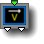12 Set Vector (Ax,Bx)

parameters :
A : A (-4. : 4.)
B : B (-4. : 4.)

discussion :
Scale the input value using parameters A and B. Left output is A*x. Right output is B*x.

##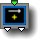12 Add (x+A,x+B)

parameters :
A : A (-50. : 50.)
B : B (-50. : 50.)

discussion :
This component is sometimes referred to as Add Vector. The outputs are x+A, x+B. Add Vector can be useful for adding offsets to the same axis or to move several objects.

##12 2D derivative (dx,dy)

parameters :
A : Amplitude (-8. : 8.)
B : Normalize % (0. : 1.)

##12 Modulo & int

parameters :
A : Real Modulo (x) (0.12 : 64.)
B : Phase (-32. : 32.)
C : Int Modulo (y) (2 : 32)

discussion :
This component provides a few different flavors of Modulo and Integer functions. The left output is a continuous floating point version of the modulo/remainder function (see
11 Modulo). The right output is the discontinuous integer modulo function (which is equivalent to the 11 Quantize component).

algorithms :
• Modulo & int Mod C:
The left-output is a continuous floating point modulo (remainder) function equivalent to 11 Modulo. The right output is a discontinuous integer-quantized modulo (equivalent to 11 Quantize ) where parameter C determines the number of steps.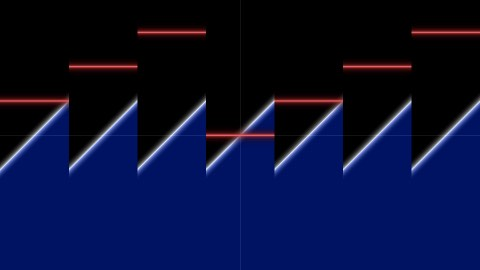Modulo & int Mod C with the int constant part (in red) looping over 4 integer values with modulo 4

• Modulo & integer part:
Left-output is the floating point (continuous) modulo. The right-output returns the integer part of the input. The parameter option provides tiling options that limit the number of steps. This output can be very useful for differentiating copies and making them behave differently.

• Modulo & Random Value:
Right-output: return a unique random value B for each step of the modulo function. The parameter option provides tiling options that limit the number of steps.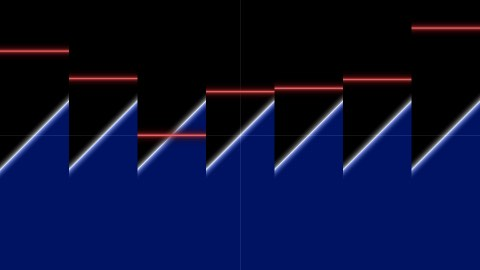With Modulo & Random Value a constant random value (in red) is associated with each ramp cycle

• Modulo & smooth step:
Similar to "Modulo & integer part" but the step function is made continuous with smoothing of the steps. The modulo returned by the x-output is the modulo of the input's absolute value and so is symmetrical. This mode was used to animate the legs in Legs Mechanics ArtMatic Voyager example. The parameter option provides tiling options that limit the number of steps.

##12 Integer Modulo

parameters :
A : Modulo (2 : 32)
B : Freq x (0. : 1.)
C : Freq y (0. : 1.)

discussion :
Integer Modulo essentially packs two 11 Quantize components into a single tile . The left and right outputs share the same number of steps but have independently controllable spacing via the frequency parameters.

##12 Cubic Clip

parameters :
A : Input Scale (0. : 2.)
B : Offset (-4. : 4.)
C : Amplitude (0. : 32.)

discussion :
The left output is the Cubic Clip function (see the 11 component description). The right output simply passes through the input value.

##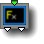12 Math tools

parameters :
A : Offset A (-50. : 50.)
B : Offset B (-50. : 50.)
C : Thickness N (0. : 10.)
D : Thickness N (0. : 10.)

discussion :
This component provides a few useful math functions selected by the algorithms popup.

algorithms :
• log(Ax + B) & exp(Ax + B):
The left output is the log and the right output is the exponential.
• exp(x s) & exp(-x s) B :slope:
Parameter B acts as a slope value. The left output returns the positive exponent and the right output the negative exponent. The two curves are symmetrical. When creating DFRM objects, the second output could be used, for example, to thin the field while the first output is being used to scale the y-axis.
• tan(x) & atan(x):
returns tan(x) in output 1 and atan(x) in output 2.
• Offset & Abs:
Provides 2 abs function with independent offsets and height. This operator does not scale space and is DF friendly.
• Offset & N-Abs
Provides 2 mirror function with independent offsets and height. This operator does not scale space and is DF friendly. N-Abs is basically the DF 2D line equation so you can think of Offset & N-Abs as a way to get 2 DF lines at once that you can position with the offsets A & B. A 2D xy DF line equation is actually a 3D DF plane, since it is infinite in z. Offset & N-Abs plot, Red curve is second output below: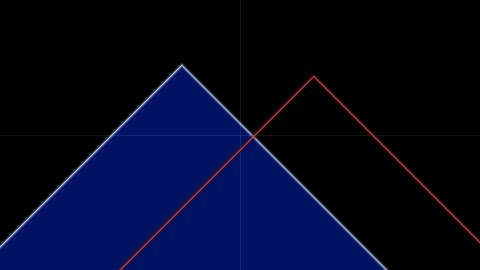##12 Linear motion

parameters :
A : Direction (angle) (-180. : 180.)
B : Speed (-50. : 50.)

##12 Circular motion

parameters :
A : Radius (0. : 100.)
B : Speed X (-10. : 10.)
C : Speed Y (-10. : 10.)

##12 Random motion

parameters :
A : Amplitude (0. : 100.)
B : Speed % (-50. : 50.)

##12 Saw motion

parameters :
A : Amplitude (-10. : 10.)

discussion :
Saw motion uses two dephased triangle saw waves to create a 45° rotated square motion. Amplitude controls both frequency and size which means this component can also be used for DF 3D modeling. This tile is available starting ArtMatic Engine 8.0. The tile has the options to interpret the Amplitude in various units.

##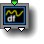12 Perlin noise + derivative

parameters :
A : Amplitude (-8. : 8.)
B : Phase (-32. : 32.)
C : Frequency (-8. : 32.)

discussion :
The left-output is a 1D Perlin Noise function with its derivative provided from the second output. Derivative can be used to rotate an animated object while the the left-output value animates its position.
Here one can see the derivative in red crossing zero whenever the perlin noise is flat, as expected :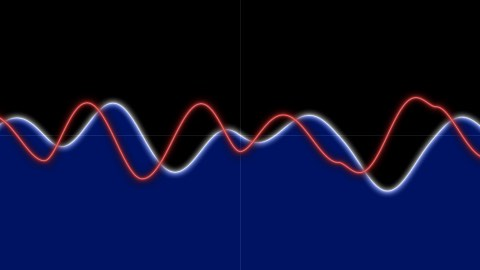## 12 Compiled Tree :

parameters :
A : Scale 0:1
B : Iterations

discussion :
Compiled Trees are groups of tiles that can be used in place of single tiles as a kind of macro or subroutine. 12 CT can hold any 1 input / 2 outputs tree and can be seen generally as a 2D vector valued 1D function.
12 CT are often used to define 2D coordinates derived from a single input (typically time). When input is time one can see the CT as the motion path equation or a 2D parametric curve of 't'. For example
x=cos(t),y=sin(t) will define a circular motion. To efficiently render parametric curve ArtMatic Engine 8 offer a new component, 2D Motion Path render, that will work in tandem with 12 Compiled trees.

usage :
Select a 12 tile and use "New compiled tree" to create a new CT from the selection (Tree Edit menu or type 'n' key).
To save a CT on disk to use the function elsewhere use "Save compiled tree" from the Tree Edit menu.
You may also copy and paste the entire CT by using Copy Tile and Paste Tile from the Edit menu.
You may iterate the tree by "Iterations" number when the tree contains an Iterations tile to modify and accumulates various values. "Scale" provide an optional scaling of outputs which is set at 1 by default (no scaling).